# Solved 2024 Specimen Paper ICSE Class 10 Physics

## Section A (40 Marks)

#### Question 1(i)

A moment of couple has a tendency to rotate the body in an anticlockwise direction then that moment of couple is taken as:

1. positive
2. negative
3. maximum
4. zero

positive

Reason — The anticlockwise moment is taken as positive while the clockwise moment is taken as negative.

#### Question 1(ii)

The kinetic energy of a given body depends on the:

1. position
2. centre of gravity of body
3. momentum
4. displacement

momentum

Reason — As kinetic energy = $\dfrac{1}{2}$ mv 2

If we multiply and divide by m then we get, $\dfrac{\text{(mv)}^2}{2\text{m}}$

As mv = momentum (p), so

kinetic energy = $\dfrac{\text{p}^2}{2\text{m}}$

Therefore, kinetic energy of a given body depends on the momentum.

#### Question 1(iii)

For burning of coal in a thermoelectric station, the energy conversion taking place is:

1. chemical to heat to mechanical
2. chemical to heat to mechanical to electrical
3. chemical to heat to light
4. heat to chemical to mechanical

chemical to heat to mechanical to electrical.

Reason — A thermal power station is a type of power station in which heat energy is converted to electrical energy.

Here, the chemical energy of coal is changed into heat energy, which is transferred to steam. Then the heat energy of steam changes into mechanical energy of turbine that is further changed into electrical energy.

#### Question 1(iv)

A nucleus of an atom consists of 146 neutrons and 95 protons. It decays after emitting an alpha particle. How many protons and neutrons are left in the nucleus after an alpha emission?

1. protons = 93, neutrons = 144
2. protons = 95, neutrons = 142
3. protons = 89, neutrons = 144
4. protons = 89, neutrons = 142

protons = 93, neutrons = 144

Reason — An alpha particle consists of two protons and two neutrons. Emission of alpha particle reduces the number of protons by 2 and also the number of neutrons by 2. Hence, number of protons = 95 - 2 = 93 and number of neutrons = 146 - 2 = 144.

#### Question 1(v)

Assertion: Infrared radiations travel long distances through dense fog and mist.

Reason: Infrared radiations undergoes minimal scattering in earth's atmosphere

1. both assertion and reason are true.
2. both assertion and reason are false.
3. assertion is false but reason is true.
4. assertion is true reason is false.

both assertion and reason are true.

Reason — Infrared radiations travel long distances through dense fog and mist as they undergo minimal scattering in earth's atmosphere.

#### Question 1(vi)

For a convex lens, the minimum distance between an object and its real image in terms of focal length (f) of a given lens must be:

1. 1.5 f
2. 2.5 f
3. 2 f
4. 4 f

4f

Reason — When the object is kept at 2F1, then, its image is formed at 2F2. This is the position of minimum distance between object and image (i.e., 2f + 2f = 4f).

#### Question 1(vii)

Two sound waves X and Y have same amplitude and same wave pattern, but their frequencies are 60 Hz and 120 Hz respectively, then:

1. X will be shriller and Y will be grave
2. X will be grave and Y will be shriller
3. X will differ in quality than Y
4. X is louder than Y.

X will be grave and Y will be shriller.

Reason — The shrillness of a sound wave is directly proportional to its frequency, meaning that higher frequencies result in shriller sounds and lower frequencies result in grave sound.

#### Question 1(viii)

Vibrations produced in a body under the influence of the periodic force is;

1. forced vibrations
2. resonant vibrations
3. damped vibrations
4. sympathetic vibrations

forced vibrations

Reason — The vibrations of a body which take place under the influence of an external periodic force acting on it, are called forced vibrations. E.g. the vibrations produced in the diaphragm of a microphone sound box with frequencies corresponding to the speech of the speaker, are the forced vibrations.

#### Question 1(ix)

The graph of voltage vs current for four different materials is shown below.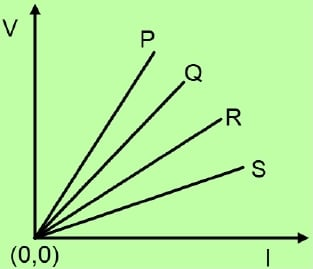Which of these four materials would be used for making the coil of a toaster?

1. Q
2. S
3. P
4. R

P

Reason — The coil of a toaster should produce a high heating effect so its resistance should be high. From the graph, slope of line for substance P is the greatest hence, resistance of P is highest. So, substance P is most suitable for making the coil of a toaster.

#### Question 1(x)

According to the old convention the color of the earth wire is:

1. black
2. green
3. yellow
4. red

green

Reason— In the old colour convention, green colour is used for earth wire.

#### Question 1(xi)

Lenz’s law is based on the law of conservation of:

1. force
2. charge
3. mass
4. energy

energy

Reason — Lenz's law is based on the law of conservation of energy. It shows that the mechanical energy is spent in doing work against the opposing force experienced by the moving magnet, and it is transformed into the electrical energy due to which current flows in the solenoid.

#### Question 1(xii)

Heat capacity of a body is:

1. the energy needed to melt the body without the change in its temperature.
2. the energy needed to raise the temperature of the body by 1°C
3. the increase in the volume of the body when its temperature increases by 1°C
4. the total amount of internal energy that is constant.

the energy needed to raise the temperature of the body by 1°C

Reason — The term heat capacity of a body is the amount of heat energy required to raise it's temperature by 1° C (or 1 K). The S.I. unit of heat capacity is joule per kelvin (J K-1)

#### Question 1(xiii)

The amount of heat energy required to melt a given mass of a substance at its melting point without rise in its temperature is called:

1. specific heat capacity
2. specific latent heat of fusion
3. latent heat of fusion
4. specific latent heat of freezing

latent heat of fusion

Reason — Heat energy absorbed in change of phase that is not externally manifested by any rise in temperature is called the latent heat of fusion.

#### Question 1(xiv)

When a ray of light enters from a denser medium to a rarer medium then:

1. the light ray bends towards the normal
2. the speed of light increases
3. the angle of incidence is greater than the angle of refraction
4. its wavelength decreases.

the speed of light increases

Reason — The speed of light increases when it enters from a denser medium to a rarer medium. Lower density of the rarer medium causes less interaction between photons and particles. This reduces the scattering and results in a higher speed.

#### Question 1(xv)

An endoscope uses optical fiber to transmit high resolution images of internal organs without loss of information. The phenomenon of light that governs the functioning of the optical fiber is:

1. refraction
2. reflection
3. scattering
4. total internal reflection

total internal reflection.

Reason — Total internal reflection is the principle that allows light to propagate through the core of an optical fiber by continuously reflecting off the inner walls of the fiber due to the angle at which it strikes those walls.

#### Question 2(i)

(a) Name the principle on which a lever works.

(b) Which radiations that are emitted during the decay of a nucleus, having highest penetrating power?

(c) Does the emission of the above-mentioned radiation result in a change in the mass number?

(a) A lever works on the principle of moments, according to which in the equilibrium position of levers, moment of load about the fulcrum must be equal to the moment of effort about the fulcrum and the two moments must be in opposite directions.

(b) Gamma radiations emitted during the decay of a nucleus have the highest penetrating power.

(c) When gamma radiations are emitted from the nucleus then there is no change in mass number as gamma radiations have no charge and mass.

#### Question 2(ii)

A metre rod made of copper and steel as shown in the diagram. Weights of copper and steel are 10 N and 8 N respectively.

(a) On which part does the centre of gravity lie (0 to 50 or 50 to 100).(a) Centre of gravity of the given rod lie between 0 to 50.

(b) In equilibrium ,

(F1) x distance (l1) = (F2) x distance (l2)

As, F1 > F2

Hence, l1 < l2

Therefore, centre of gravity lies between 0 to 50.

#### Question 2(iii)

A lever is shown below.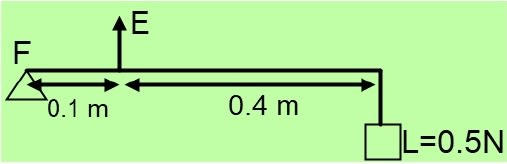(a) Identify the type of lever.

(a) It is a class III lever.

(b) Mechanical advantage = $\dfrac{\text{effort arm}}{\text{load arm}}$ = $\dfrac{0.1}{0.4}$ = $\dfrac{1}{4}$ = 0.25

#### Question 2(iv)

Two bodies A and B have same kinetic energies. Compare their velocities if mass of A is four times the mass of B.

Considering Body A:

Let mass of body A = ma = 4m

Let kinetic energy of body A = K

Let velocity of body A = Va

Considering Body B:

Mass of body B = mb = m [∵ mass of A is four times the mass of B]

Kinetic energy of body B = K [∵ A and B have same kinetic energies]

Let velocity of body B = Vb

Given, A and B have same kinetic energies,

$\dfrac{\text{1}}{\text{2}}$ 4mVa2 = $\dfrac{\text{1}}{\text{2}}$mVb2

4mVa2 = mVb2

$\dfrac{\text{V}_a^2}{\text{V}_b^2} = \dfrac{\text{1}}{\text{4}} \\[1em] \Rightarrow \dfrac{\text{V}_a}{\text{V}_b} = \sqrt{\dfrac{\text{1}}{\text{4}}} \\[1em] \Rightarrow \dfrac{\text{V}_a}{\text{V}_b} = \dfrac{1}{2}$

Hence, Va : Vb = 1 : 2 or velocity of body B is two times the velocity of body A.

#### Question 2(v)

Draw a graph of potential energy vs height from the ground for a body thrown vertically upwards.

Graph of potential energy vs height from the ground for a body thrown vertically upwards is shown below: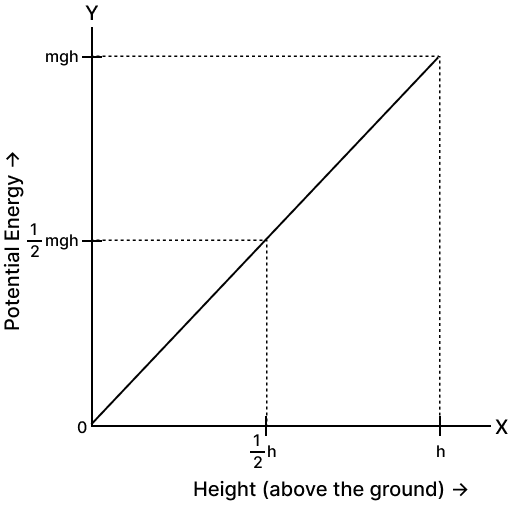#### Question 2(vi)

Two copper wires A and B are of the same thickness and are at the room temperature. If the length of A is twice the length of B then :

(a) Compare their resistances

(b) Compare their resistivity

(a) Let the length of wire B be L and that of A be 2L

R= ρ$\dfrac{\text{length}}{\text{area}}$

As area and specific resistance (ρ) are same for both wires A and B,

Hence,

$\dfrac{\text{R}_a}{\text{R}_b}$ = $\dfrac{\text{l}_a}{\text{l}_b}$ = $\dfrac{\text{2L}}{\text{L}}$ = $\dfrac{\text{2}}{\text{1}}$

Hence, the ratio between resistances A and B is 2 : 1

(b) Both the wires will have the same specific resistance because the specific resistance is a characteristic property of the material and as both the wires are of copper hence both will have same specific resistance.

#### Question 2(vii)

(a) Name the waves used for echo depth sounding.

(b) Give one reason for their use in the above application

(a) The waves used for echo depth sounding are ultrasonic waves.

(b) Ultrasonic waves can travel undeviated through a long distance and so they are used for echo depth sounding.

#### Question 3(i)

(a) Refer to the diagram given below. A lens with two different refractive indices is shown. If the rays are coming from a distant object, then how many images will be seen?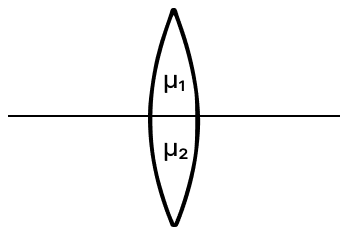(b) A glass lens always forms a virtual, erect and diminished image of an object kept in front of it. Identify the lens.

(a) Two images will be seen.
Reason — According to the lens maker formula :

$\dfrac{1}{\text{f}}$ = (μ - 1)($\dfrac{1}{\text{R}_1}$ - $\dfrac{1}{\text{R}_2}$)

Focal length is a function of refractive index, hence for two different refractive index there will be two different focal lengths.

Two different focal length implies two different images for same object. Hence 2 images will be formed.

(b) Concave lens

#### Question 3(ii)

It is observed that the house circuits are arranged in a parallel combination. Give two advantages of this arrangement.

(a) Two advantages of this arrangement are :

1. Each appliance operates independently without being affected by the presence or working of the other appliances.
2. Each appliance gets connected to 220 V supply (= its voltage rating) for its normal working.

#### Question 3(iii)

A transformer is used to change a high alternating e.m.f. to a low alternating e.m.f. of the same frequency.

(a) Identify the type of transformer used for the above purpose.

(b) State whether the turns ratio of the above transformer is = 1 or >1 or <1.

(a) Step down transformer

(b) turns ratio is n < 1, i.e., number of turns in the secondary are less than the number of turns in the primary.

#### Question 3(iv)

A solid of mass 60 g at 100°C is placed in 150 g of water at 20°C. The final steady temperature is 25°C. Calculate the heat capacity of solid?

[sp. heat capacity of water = 4.2 J g-1 K-1 ]

Given,

Mass of solid (m) = 60 g

Fall in temperature of solid = (100 - 25) = 75°C

Rise in temperature of water = (25 - 20) = 5°C

Let specific heat capacity of solid be c.

Heat energy given by solid = mc△t
= 60 x c x 75 = 4500 x c .....(1)

Heat energy taken by water = 150 × 4.2 × 5 = 3150 .....(2)

Assuming that there is no loss of heat energy,

Heat energy given by solid = Heat energy taken by water.

Equating equations 1 & 2, we get,

$4500 \times c = 3150 \\[0.5em] c = \dfrac{3150}{4500} \\[0.5em] c = 0.7 \text{ J g}^{-1} \text{K}^{-1} \\[0.5em]$

specific heat capacity of the solid = 0.7 J g-1 K-1

Heat capacity of solid = mass (m) x specific heat capacity = 60 x 0.7 = 42 J K-1

Hence, Heat capacity of solid = 42 J K-1

#### Question 3(v)

What is a nuclear waste? State one method to dispose it safely.

The radioactive material after its use such as nuclear power generation, nuclear weapons production, and nuclear medicine is known as nuclear waste.

The nuclear waste obtained from laboratories, hospitals, scientific establishments or power plants must be first kept in thick casks and then they must be buried in the specially constructed deep underground stores. These underground stores must be far away from the populated areas.

## Section B (40 Marks)

#### Question 4(i)

The diagram below shows a fish in the tank and its image seen in the surface of water.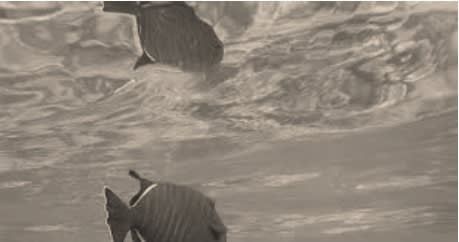(a) Name the phenomenon responsible for the formation of this image.

(b) Complete the path of the ray through the glass prism of critical angle 42° till it emerges out of the prism.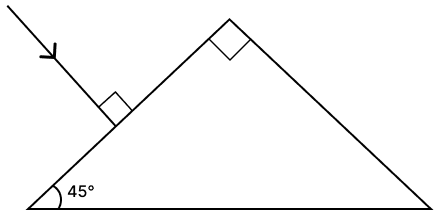(a) Refraction

(b) Below diagram shows the completed path of the ray till it emerges out of the prism: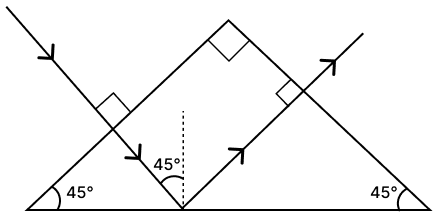#### Question 4(ii)

(a) The refractive index of water is 1.33 at a certain temperature. When the temperature of water is increased by 40°C, the refractive index changes to 'x'. State whether x < 1.33 or x > 1.33.

(b) State two differences between normal reflection and total internal reflection.

(a) In general, the refractive index of a substance decreases with increasing temperature. Therefore, if the temperature of water is increased by 40°C, the refractive index 'x' is expected to be lesser than 1.33. Hence, we can state that x < 1.33.

(b) Two differences between normal reflection and total internal reflection are:

S.
No.
Normal reflectionTotal internal reflection
1.Normal reflection can occur whenever light encounters a reflective surface, such as a mirror, glass, or water, without any specific conditions related to the angle of incidence.Total internal reflection occurs when light travelling in a denser medium, is incident at the surface of a rarer medium at an angle of incidence greater than the critical angle for the pair of media.
2.In normal reflection, only a part of the light is reflected while rest is refracted and absorbed. So, the reflection is partial.In total internal reflection, the entire incident light is reflected back into the denser medium. So there is no loss of light energy.

#### Question 4(iii)

The below diagram shows that an observer sees the image of an object O at I.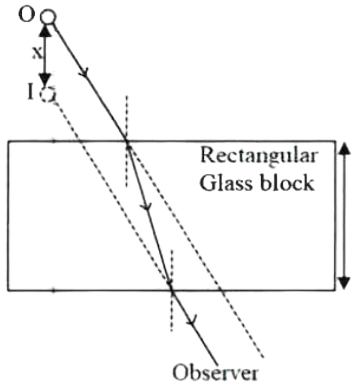(a) Name and define the phenomenon responsible for seeing the image at a different position.

(b) State the effect on X when:

1. Y increases
2. Y decreases

(a) Refraction

The change in direction of the path of light when it passes from one transparent medium to another transparent medium, is called refraction.

(b) The shift X,

1. increases when Y increases
2. decreases when Y decreases.

#### Question 5(i)

An object is placed at a distance 24 cm in front of a convex lens of focal length 8 cm.

(a) What is the nature of the image so formed?

(b) Calculate the distance of the image from the lens.

(a) Real and inverted image is formed.

(b) Given, focal length = 8 cm

Object distance (u) = -24 cm

Image distance (v) = ?

From lens formula —

$\dfrac{1}{v} - \dfrac{1}{u} = \dfrac{1}{f} \\[1em]$

Substituting the values in the formula we get,

$\dfrac{1}{\text{v}} - \dfrac{1}{-24} = \dfrac{1}{8} \\[1em] \dfrac{1}{\text{v}} + \dfrac{1}{24} = \dfrac{1}{8} \\[1em] \dfrac{1}{\text{v}} = \dfrac{1}{8} - \dfrac{1}{24}\\[1em] \Rightarrow \dfrac{1}{\text{v}} = \dfrac{3 - 1}{24} \\[1em] \Rightarrow \dfrac{1}{\text{v}} = \dfrac{2}{24} \\[1em] \Rightarrow \dfrac{1}{\text{v}} = \dfrac{1}{12} \\[1em] \Rightarrow v = 12$

∴ The image is formed at a distance of 12 cm behind the lens.

#### Question 5(ii)

When sunlight passes through water droplets in the atmosphere it gets dispersed into its constituent colours forming a rainbow. A similar phenomenon is observed when white light passes through a prism.

(a) Which colour will show the maximum angle of deviation and which colour will show the minimum angle of deviation?

(b) If instead of sunlight, a green-coloured ray is passed through a glass prism. What will be the colour of the emergent ray?

(a) When sunlight passes through water droplets in the atmosphere or through a prism, violet light shows the maximum angle of deviation, while red light shows the minimum angle of deviation.

(b) The colour of emergent ray will be green. A prism does not change the colour of light. It just disperses white light into its constituent colours.

#### Question 5(iii)

(a) Mixture of red + blue + green is passed through a convex lens as shown in the diagram below. State whether the ray passes through a single point or through different points on principle axis after refraction.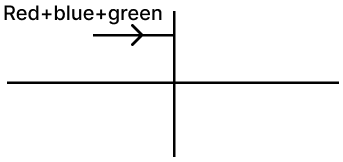(b) Name the invisible radiations which can be obtained using quartz prism? State one use of these radiations.

(c) Name one radiations having wavelength longer than the wavelength of these radiations.

(a) The ray will pass through different points on principle axis after refraction through the convex lens. Red colour will deviate the least, followed by green and then blue will deviate the most.

(b) Ultraviolet radiations. Ultraviolet radiations are used for sterilising air, surgical equipment, etc.

#### Question 6(i)

Sumit and Sachin went for a trek and during the journey they visited a cottage. They suspended their bags to the two ropes hanging from P and Q on a wheel capable of rotating around O. Sumit suspended his bag to the rope Q and Sachin suspended his bag from the rope P. The wheel remained in equilibrium.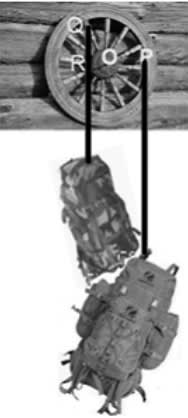(a) State with a reason who is carrying a heavier bag.

(b) Based on the principle of moments, write a mathematical relation that can be used to determine the weight (W) of Sachin's bag, given that the weight of Sumit's bag is 18 kgf.

(a) At equilibrium clockwise moment = anticlockwise moment

Moment = force x perpendicular distance

Wt. of Sachin's bag x OP = Wt. of Sumit's bag x OR

As OR < OP. Hence, Wt. of Sumit's bag > Wt. of Sachin's bag.

Hence, Sumit's bag is heavier than Sachin's bag

(b) Given,

Wt. of Sumit's bag = 18 kgf

Let wt. of Sumit's bag be W

At equilibrium,

Clockwise moment = Anticlockwise moment

W x OP = 18 x OR

W = $\dfrac{18 \times \text{OR}}{\text{OP}}$

#### Question 6(ii)

The diagram below shows a block and tackle system.(a) Copy and complete the labelled diagram showing the correct connection of the tackle, the direction of the forces involved to obtain maximum V.R. with the convenient direction.

(b) Calculate the M.A. of this pulley system if its efficiency is 80%.

(a) The completed diagram is given below: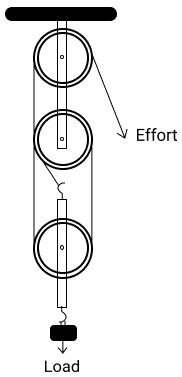(b) η = 80% given

V.R. = n = 3,

M.A. =?

η = $\dfrac{\text{M.A.}}{\text{V.R.}}$

$\dfrac{\text{80}}{\text{100}}$ = $\dfrac{\text{M.A.}}{\text{3}}$

M.A. = $\dfrac{\text{80}}{\text{100}}$ x 3= $\dfrac{\text{240}}{\text{100}}$ = 2.4

#### Question 6(iii)

The figure below shows a simple pendulum of mass 200 g. It is displaced from the mean position A to the extreme position B. The potential energy at the position A is zero. At the position B the pendulum bob is raised by 5 m.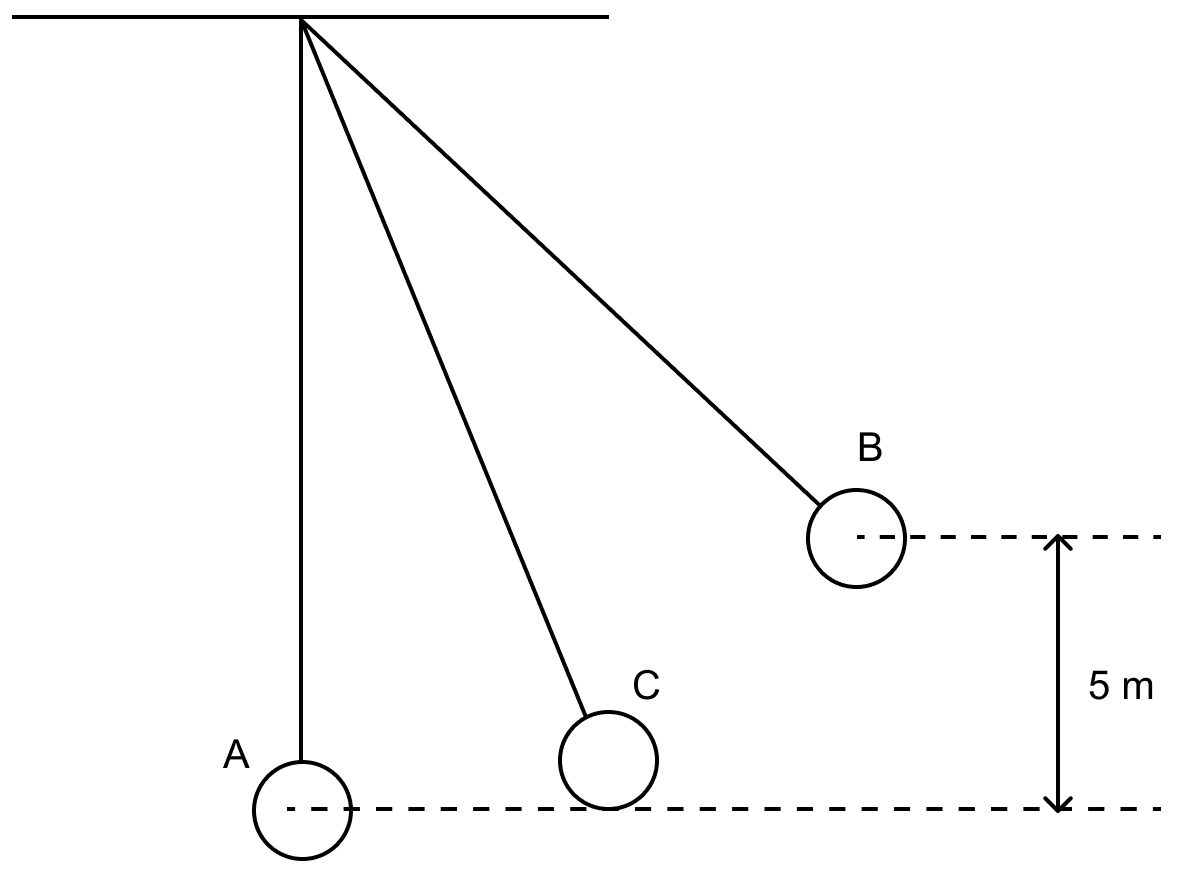(a) What is the potential energy of the pendulum at the position B?

(b) What is the total mechanical energy at point C?

(c) What is the speed of the bob at the position A when released from B?

(Take g = 10 ms-2 and given that there is no loss of energy.)

Given,

h = 5 m

m = 200 g = 0.2 kg

g = 10 ms-2

(i) Potential energy UB at B is given by

UB = m x g x h

Substituting the values we get,

UB = 0.2 x 10 x 5 = 10 J

Hence, the potential energy of the pendulum at the position B = 10 J

(ii) Total mechanical energy at point C = 10 J

The total mechanical energy is same at all points of the path due to conservation of mechanical energy.

(iii) At A, bob has only kinetic energy which is equal to potential energy at B,

Therefore,

$\dfrac{1}{2} \times m \times (v_A)^{2} = U_B \\[0.5em] 0.5 \times 0.2 \times (v_A)^{2} = 10 \\[0.5em] (v_A)^{2} = \dfrac{10}{0.1} \\[0.5em] \Rightarrow v_A = \sqrt{100} \\[0.5em] \Rightarrow v_A = 10 \text{ m s}^{-1}$

#### Question 7(i)

A person standing in front of a cliff fires a gun and hears its echo after 3s. If the speed of sound in air is 336 ms-1

(a) Calculate the distance of the person from the cliff.

(b) After moving a certain distance from the cliff, he fires the gun again and this time the echo is heard 1.5 s later than the first. Calculate distance moved by the person.

(a) Let the distance of the cliff from the initial position of the person be x m.

Time after which echo is heard = 3 sec

So, the distance travelled by sound in 3 sec = 2x m

Speed of sound in air = 336 m/s

Distance = speed x time

Substituting the values we get,

$2x = 336 \times 3 \\[1em] \Rightarrow x = \dfrac{336 \times 3}{2} \\[1em] \Rightarrow x = 168 \times 3 \\[1em] \Rightarrow x = 504 \text{ m}$

∴ The initial distance of the person from the cliff is 504 m.

(b) As time taken to hear the echo is increased by 1.5 sec, hence, the person has moved away from the cliff.

Let the distance moved by the person from his initial position be y m.

Time after which echo is heard = 1.5 + 3 = 4.5 sec

New distance = 504 + y

So, the distance travelled by sound in 4.5 sec = 2(504 + y) m

Speed of sound in air = 336 m/s

Distance = speed x time

Substituting the values we get,

$2(504 + y) = 336 \times 4.5 \\[1em] \Rightarrow 504 + y = \dfrac{336 \times 4.5}{2} \\[1em] \Rightarrow y = (168 \times 4.5) - 504 \\[1em] \Rightarrow y = 756 - 504 \\[1em] \Rightarrow y = 252 \text{ m}$

∴ Distance moved by the person = 252 m

#### Question 7(ii)

A radioactive nucleus X emits an alpha particle followed by two beta particles to form nucleus Y.

(a) With respect to the element X, where would you position the element Y in the periodic table?

(b) What is the general name of the element X and Y.

(c) If the atomic number of Y is 80 then what is the atomic number of X?

(a) AZX ⟶ A-4Z-2X1 + 42He ⟶ A-4Z-1X2 + 0-1e ⟶ A-4ZY + 0-1e

We can see from the above that X and Y have same atomic number. Hence, X and Y will occupy the same position in the periodic table.

(b) Isotope.

(c) When the atomic no of Y = 80 and X and Y are isotopes then atomic no. of X will also be 80.

#### Question 7(iii)

A boy tunes a radio channel to a radio station 93.5 MHz.

(a) Name and define the scientific wave phenomenon involved in tuning the radio channel.

(b) Name the important characteristics of sound that is affected during this phenomenon.

(c) Convert 93.5 MHz to SI unit.

(a) Resonance
Definition — When the frequency of the externally applied periodic force on a body is equal to its natural frequency, the body readily begins to vibrate with an increased amplitude. This phenomenon is known as resonance.

(b) Loudness, when resonance occurs the loudness increases as the amplitude of the vibration is increased.

(c) 1 MHz = 106 Hz
93.5 MHz = 93.5 x 106 Hz = 9.35 x 107 Hz

#### Question 8(i)

Purvi's friend Tim wants to connect a fuse to his oven. He wants to control the oven from two different locations. Shown below is his circuit diagram.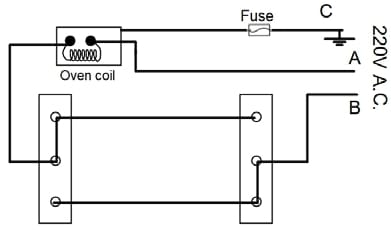(a) Which one of the two, A or B should be a live wire?

(b) In the event of an overload, will the fuse serve its purpose?

(c) What is the meaning of the statement that the bulb is rated 600W, 220 V?

(a) The switch is always connected to the live wire. In above diagram, wire B is connected to switch so wire B must be the live wire.

(b) No, the fuse will not serve its purpose. In the diagram, the fuse is connected to the earth wire and not the live wire. In case of overload, the excess current will first pass through the oven damaging it and after that will flow through the fuse.

(c) It means that if the bulb is operated on a 220 V supply, the electric power consumed by it is 600 W i.e., 600 J of electric energy is consumed by the bulb in 1 s.

#### Question 8(ii)

(a) Copy and complete the following nuclear reaction.

22286Rn ⟶ 21884Po + _—X

(b) What will be the effect on the radiation X, emitted in the above reaction when it is allowed to pass through an electric field?

(a) 22286Rn ⟶ 21884Po + 42X

(b) Radiation X are alpha particles. So, they will be deflected towards the negative plate when passed through an electric field.

#### Question 8(iii)

Observe the given circuit diagram and answer the questions that follow: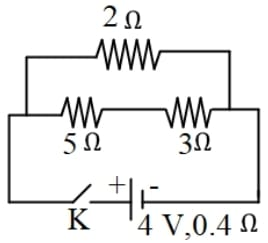(a) Calculate the resistance of the circuit when the key K completes the circuit.

(b) Calculate the current through 3Ω resistance when the circuit is complete.

(a) 5Ω and 3Ω are in series so their equivalent resistance (Rs) = 5 + 3 = 8Ω

Now 2Ω and Rs are in parallel.

$\dfrac{\text{1}}{\text{R}_\text{p}} = \dfrac{\text{1}}{\text{R}_\text{s}} + \dfrac{\text{1}}{\text{2}} \\[1em] \Rightarrow \dfrac{\text{1}}{\text{R}_\text{p}} = \dfrac{\text{1}}{\text{2}} + \dfrac{\text{1}}{\text{8}} \\[1em] \Rightarrow \dfrac{\text{1}}{\text{R}_\text{p}} = \dfrac{4+1}{8} \\[1em] \Rightarrow \dfrac{\text{1}}{\text{R}_\text{p}} = \dfrac{5}{8} \\[1em] \therefore \text{R}_\text{p} = \dfrac{8}{5} = 1.6 \space Ω\\[1em]$

From circuit diagram,

Internal Resistance = 0.4 Ω

Total resistance of circuit = 1.6 + 0.4 = 2 Ω

(b) Current drawn from the battery

$\text{I} = \dfrac{\text{e.m.f}}{\text{Total Resistance}} \\[1em] = \dfrac{4}{2} = 2 \text{ A}$

Now, the current I divides in 2 parts.

Let current across 2Ω be I1 and across the series combination of 5Ω and 3Ω be I2

I = I1 + I2 = 2 A .....(1)

Terminal Voltage of cell V = IRp = 2 x 1.6 = 3.2 V

∴ P.d. across 2 Ω resistor = 3.2 V

I1 = $\dfrac{3.2 \text{ V}}{2 \text{ Ω}}$

= 1.6 A

Substituting value of I1 in Eq 1,

2 = 1.6 + I2

⇒ I2 = 2 - 1.6 = 0.4 A

Hence, current across 3Ω = 0.4 A

#### Question 9(i)

What mass of ice at 0°C added to 2.1 kg water, will cool it down from 75°C to 25°C?

Given: Specific heat capacity of water = 4.2 Jg-1 C-1

Specific latent heat of ice = 336 Jg-1

Given,

mw = 2.1 kg = 2100 g

Specific heat capacity of water = 4.2 Jg-1 C-1

Specific latent heat of ice = 336 Jg-1

mi = ?

Heat energy given out by water in lowering it's temperature from 75° C to 25° C
= m x c x change in temperature
= 2100 x 4.2 x (75 - 25)
= 2100 x 4.2 x 50
= 4,41,000

Heat energy taken by m kg ice to melt into water at 0° C
= mi x L
= mi x 336

Heat energy taken by water at 0° C to raise it's temperature to 25° C
= mi x c x change in temperature
= mi x 4.2 x (25 - 0)
= mi x 4.2 x 25
= mi x 105

heat energy released = heat energy taken

Substituting the values we get,

4,41,000 = (mi x 336) + (mi x 105)

⇒ 4,41,000 = 336mi + 105mi

⇒ 4,41,000 = 441mi

⇒ mi = $\dfrac{441000}{441}$

⇒ mi = 1000 g or 1 Kg

Hence, the mass of ice added = 1 Kg

#### Question 9(ii)

The diagram below shows a cooling curve for a substance:

(a) State the temperatures at which the substance condenses.

(b) The temperature range in which the substance is in liquid state.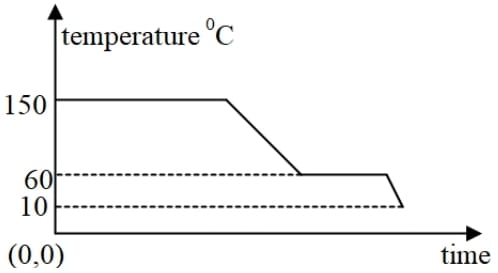(c) Why do we prefer ice to ice-cold water for cooling a drink?

(a) Substance condenses at 150° C

(b) 150 to 60°C

(c) 1 g of ice at 0° C takes 336 J of heat energy from the drink to melt into water at 0° C. Thus, the drink liberates an additional 336 J of heat energy to 1 g ice at 0° C than to 1 g ice-cold water at 0° C. Therefore, cooling produced by 1 g ice at 0° C is much more than that by 1 g water at 0° C.

#### Question 9(iii)

A magnet is released along the axis of a copper coil as shown in the diagram.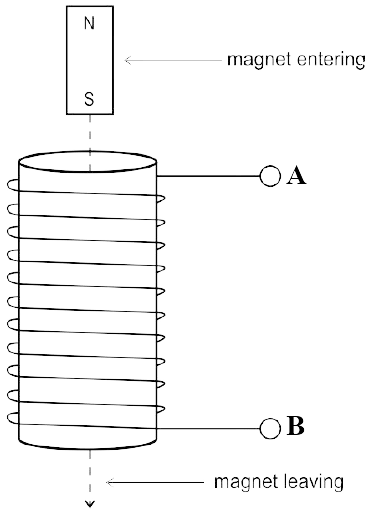(a) State the polarity at the top end of the coil when the magnet leaves the coil.

(b) The direction of the current is from A to B when magnet enters the coil. What will be the direction of the current when the magnet leaves the coil.

(c) Name the law which can be used to determine the direction of the induced current in the coil?

(d) State one way to increase the magnitude of the induced current in the coil?